# GEOMETRIC TRANSFORMATIONS Yingcai Xiao Roadmap to Geometric Transformations

• Slides: 33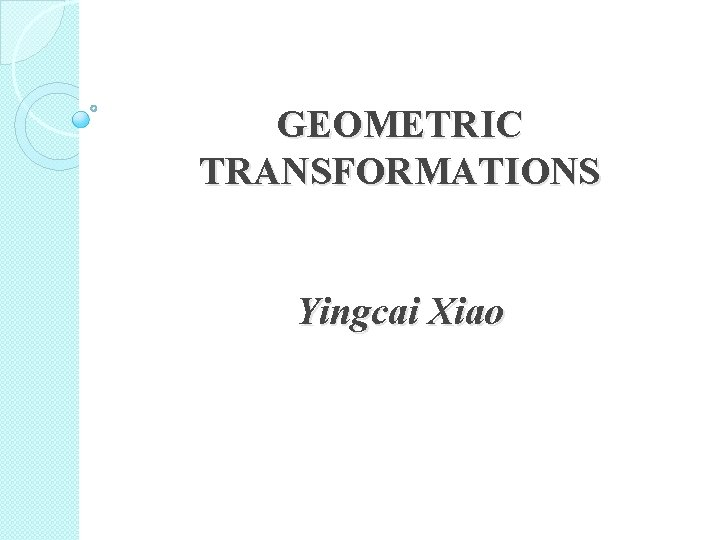GEOMETRIC TRANSFORMATIONS Yingcai XiaoRoadmap to Geometric Transformations �Start with 2 D GT �Extend to 3 D GT �Preview mathematics �Express GT in four type of notations ◦ ◦ Algebraic Matrix Logical Visual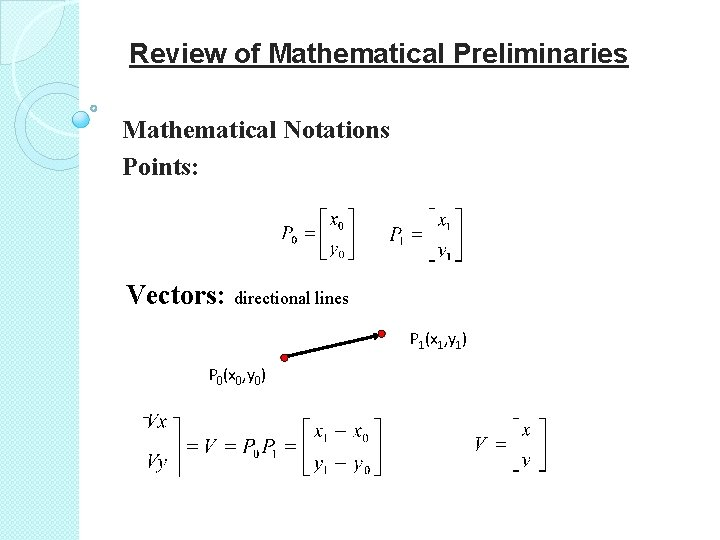Review of Mathematical Preliminaries Mathematical Notations Points: Vectors: directional lines P 1(x 1, y 1) P 0(x 0, y 0)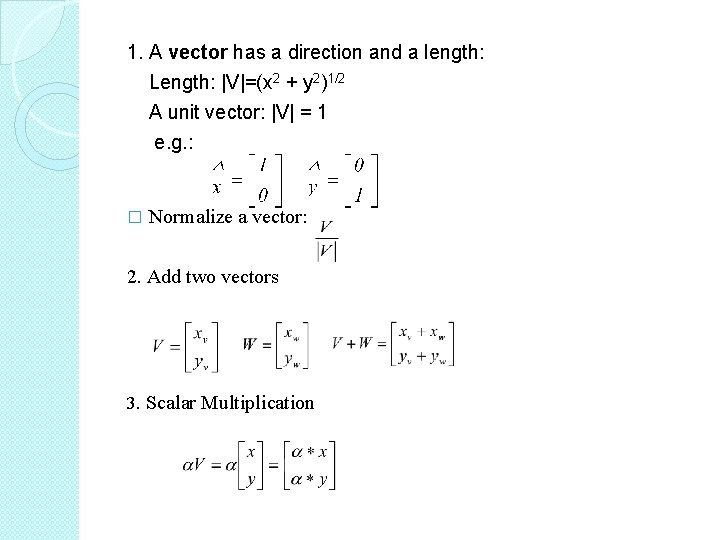1. A vector has a direction and a length: Length: |V|=(x 2 + y 2)1/2 A unit vector: |V| = 1 e. g. : � Normalize a vector: 2. Add two vectors 3. Scalar Multiplication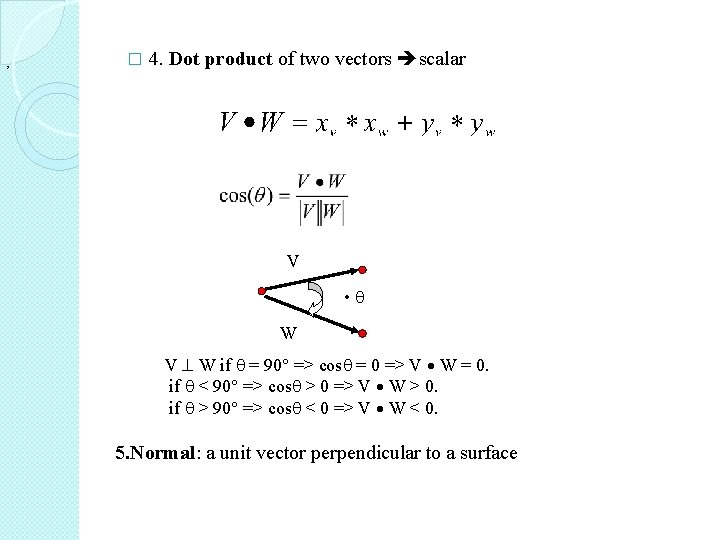� , 4. Dot product of two vectors scalar V • W V W if = 90 => cos = 0 => V W = 0. if < 90 => cos > 0 => V W > 0. if > 90 => cos < 0 => V W < 0. 5. Normal: a unit vector perpendicular to a surface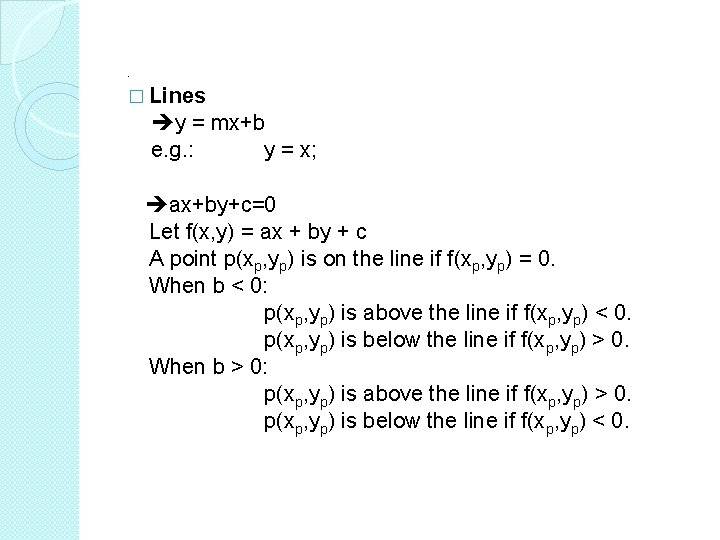. � Lines y = mx+b e. g. : y = x; ax+by+c=0 Let f(x, y) = ax + by + c A point p(xp, yp) is on the line if f(xp, yp) = 0. When b < 0: p(xp, yp) is above the line if f(xp, yp) < 0. p(xp, yp) is below the line if f(xp, yp) > 0. When b > 0: p(xp, yp) is above the line if f(xp, yp) > 0. p(xp, yp) is below the line if f(xp, yp) < 0.Parametric Form: P(t)=P 0+t(P 1 -P 0); 0 <= t <= 1 P 1(x 1, y 1) P 0(x 0, y 0) P(t)=P 0+t(P 1 -P 0)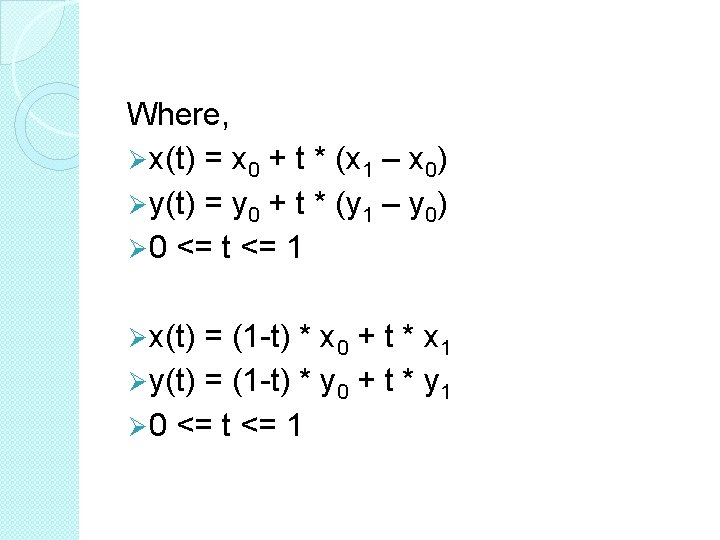� 2 D Transformations � Translate a point The algebraic representation of translation of point P(x, y) by D(dx, dy) is x’= x + dx y’= y + dy Its “matrix” representation is Its logical representation is P’ = P + D Its visual representation is P’ (x’, y’) P(x, y) D(dx, dy)� To move a shape: translate every vertex of the shape Y Y 10 10 5 5 (4, 5) (7, 5) (10, 1) (7, 1) 0 5 Before Translation 10 X 0 5 10 After Translation X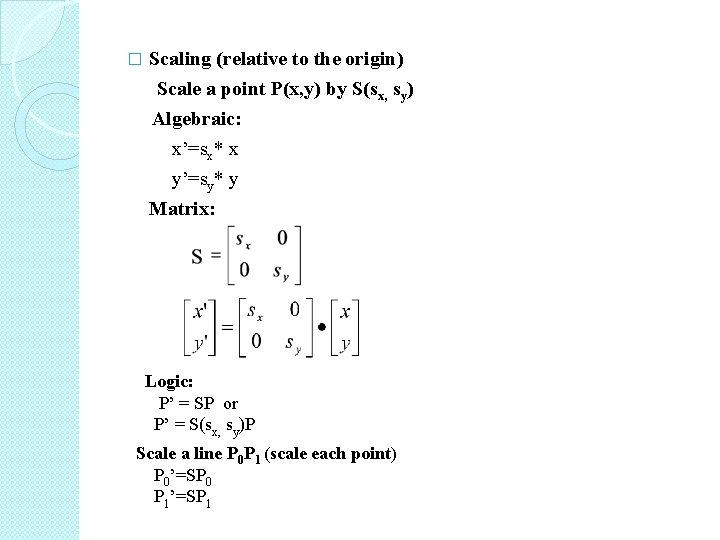Scaling (relative to the origin) Scale a point P(x, y) by S(sx, sy) Algebraic: x’=sx* x � y’=sy* y Matrix: Logic: P’ = SP or P’ = S(sx, sy)P Scale a line P 0 P 1 (scale each point) P 0’=SP 0 P 1’=SP 1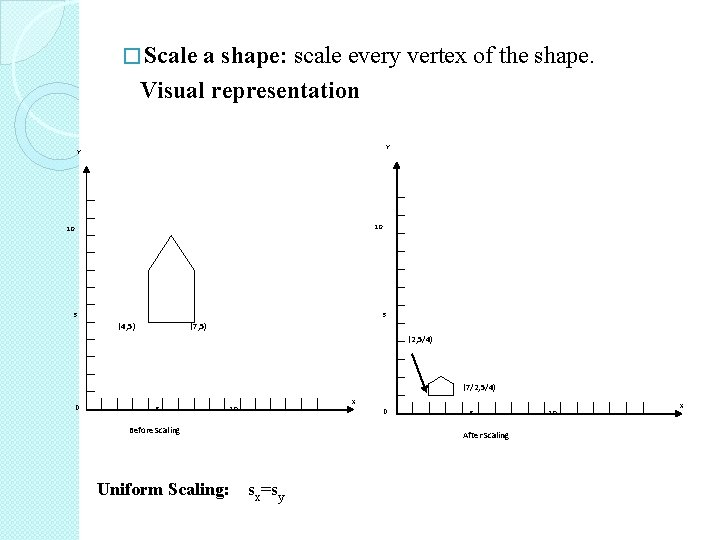� Scale a shape: scale every vertex of the shape. Visual representation Y Y 10 10 5 5 (4, 5) (7, 5) (2, 5/4) (7/2, 5/4) 0 5 X 10 0 Before Scaling Uniform Scaling: 5 After Scaling sx=sy 10 X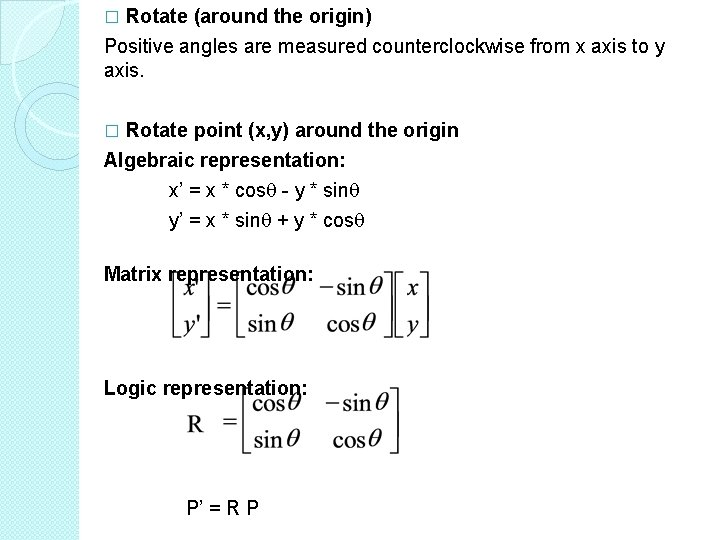Rotate (around the origin) Positive angles are measured counterclockwise from x axis to y axis. � Rotate point (x, y) around the origin Algebraic representation: x’ = x * cos - y * sin y’ = x * sin + y * cos � Matrix representation: Logic representation: P’ = R P�Visual representation Y Y 10 10 5 5 0 5 X 10 0 5 Before X 10 After Rotate a shape: rotate every vertex of the shape Y Y 10 10 (4. 9, 7. 8) 5 5 (2. 1, 4. 9) (5, 2) 0 (9, 2) 5 10 Before Rotation X 0 5 After Rotation 10 X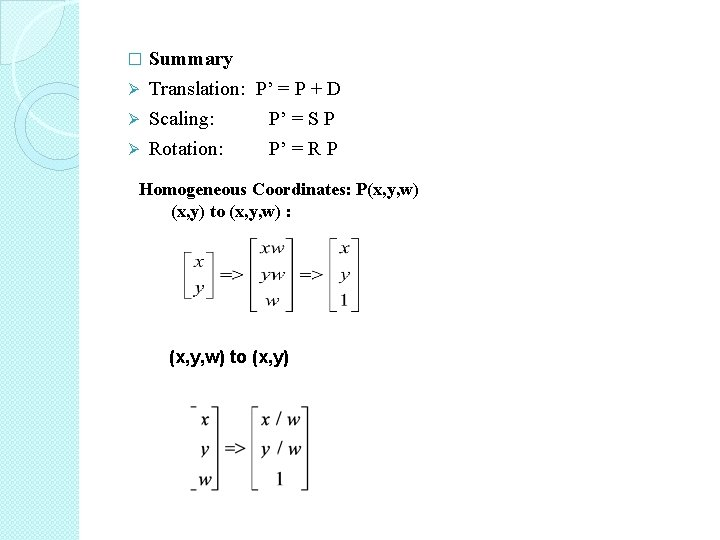Summary Ø Translation: P’ = P + D Ø Scaling: P’ = S P Ø Rotation: P’ = R P � Homogeneous Coordinates: P(x, y, w) (x, y) to (x, y, w) : (x, y, w) to (x, y)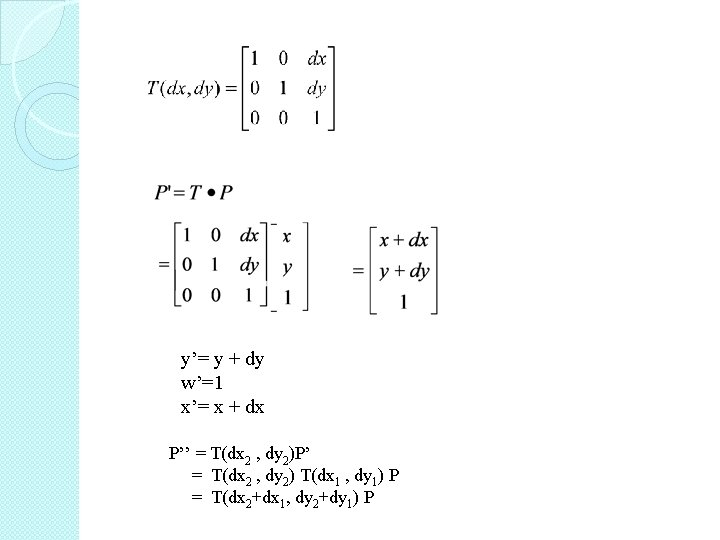y’= y + dy w’=1 x’= x + dx P’’ = T(dx 2 , dy 2)P’ = T(dx 2 , dy 2) T(dx 1 , dy 1) P = T(dx 2+dx 1, dy 2+dy 1) P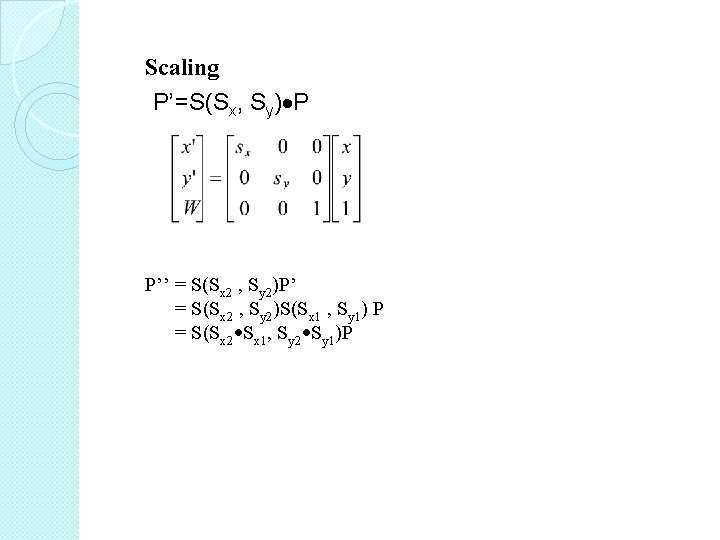Scaling P’=S(Sx, Sy) P P’’ = S(Sx 2 , Sy 2)P’ = S(Sx 2 , Sy 2)S(Sx 1 , Sy 1) P = S(Sx 2 Sx 1, Sy 2 Sy 1)P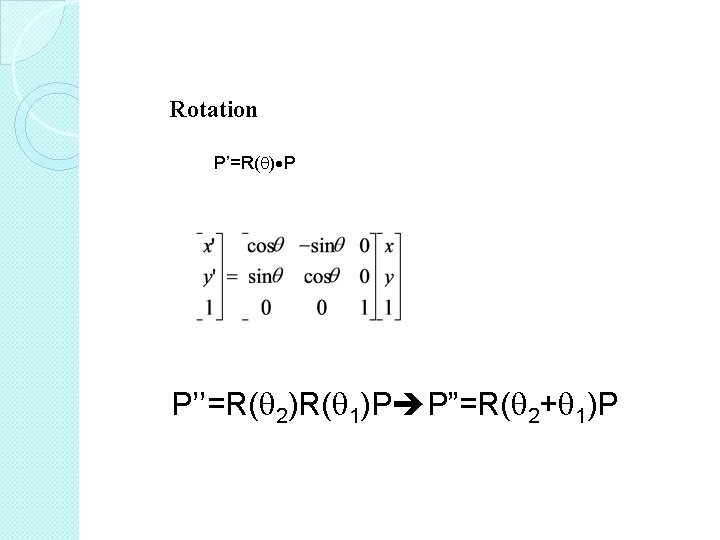Rotation P’=R( ) P P’’=R( 2)R( 1)P P”=R( 2+ 1)P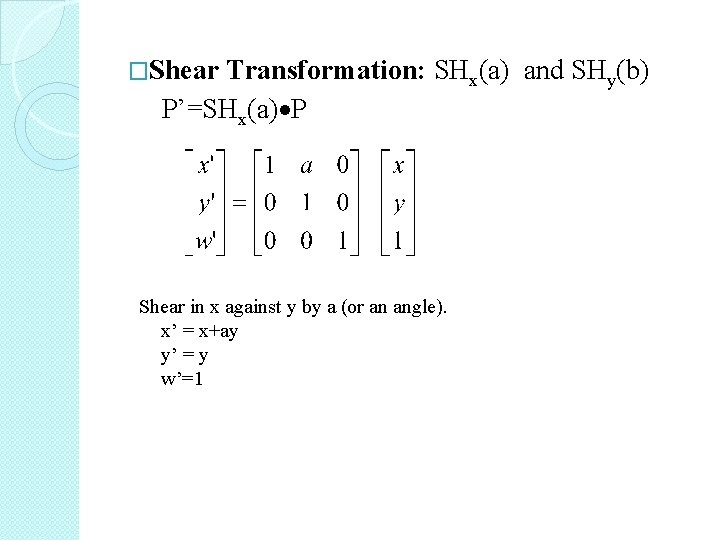�Shear Transformation: SHx(a) and SHy(b) P’=SHx(a) P Shear in x against y by a (or an angle). x’ = x+ay y’ = y w’=1P’=SHy(b) P Shear in y against x by b (or an angle). x’=x y’=y + bx w’=1.Y Y Y 10 10 10 5 5 5 0 5 10 Before Shear X 0 5 10 Sheared in x X 0 5 10 Sheared in y XRigid-body Transformation: T and R. � change: location, orientation; � not change: size, angle between elements. Affine Transformation: S, SH � change: size, location, angle; � not change: line (parallelism). � Note: uniform scaling is between the two. It changes size but not angle. � So far, our S, R, SH are all around the origin.�Composition of 2 D Transformation Rotate the house around P 1 For every vertex (P) on the object: P’ = T(P 1)R( )T(-P 1)P y y x y y After translation of P 1 to origin P 1 After rotation x x After translation To P 1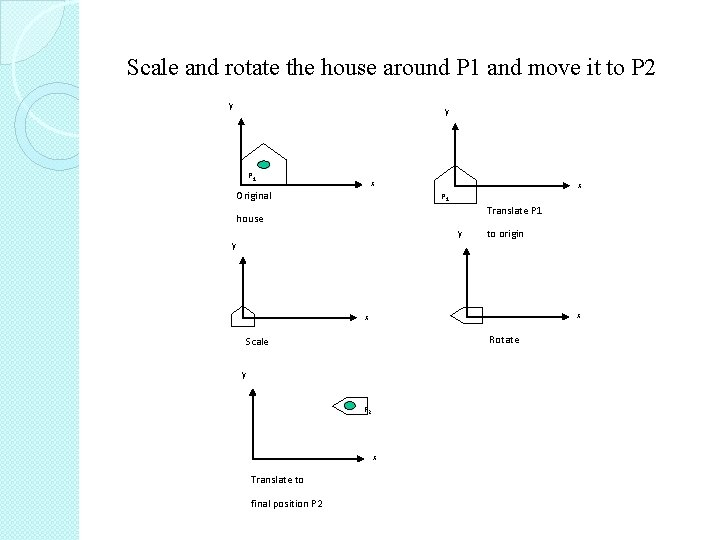Scale and rotate the house around P 1 and move it to P 2 y y P 1 x Original x P 1 Translate P 1 house y to origin y x x Rotate Scale y P 2 x Translate to final position P 2�For every vertex (P) on the object: P’ = T(P 2) R( )S(Sx, Sy)T(-P 1)P. �Swap the order of operations: not permitted, except uniform scaling (sx=sy) can be swapped with rotation.Window-to-Viewport Transformation world-coordinate: inches, feet etc & screen-coordinate: pixels y y Window World coordinates Window x Screen coordinates x y y Viewport 2 Window World coordinates x Viewport 1 x Screen coordinates Window in Window translated world coordinates to origin Maximum range of screen coordinates Window scale to size of viewport Translated by (u, v) to final position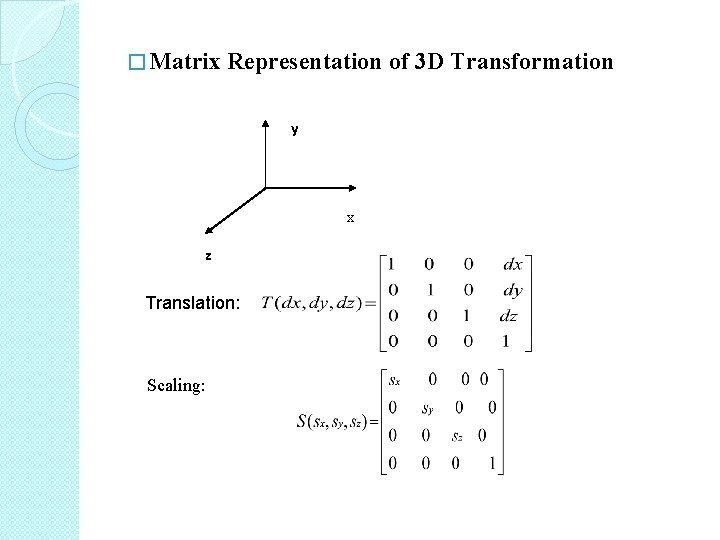� Matrix Representation of 3 D Transformation y x z Translation: Scaling: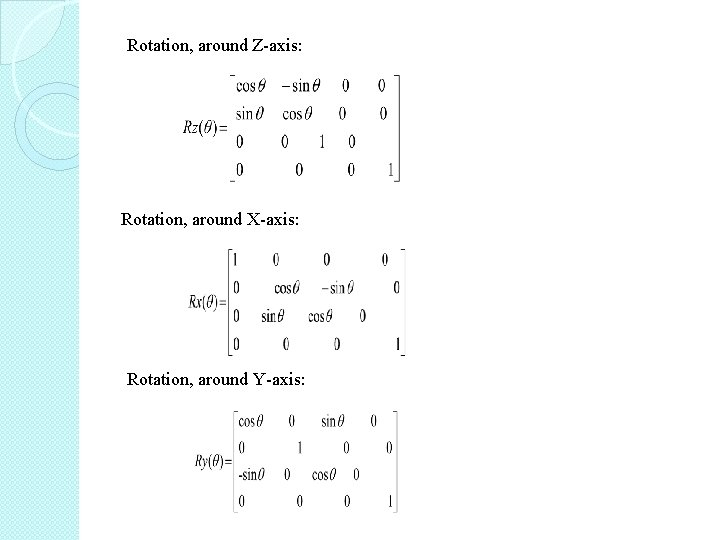Rotation, around Z-axis: Rotation, around X-axis: Rotation, around Y-axis: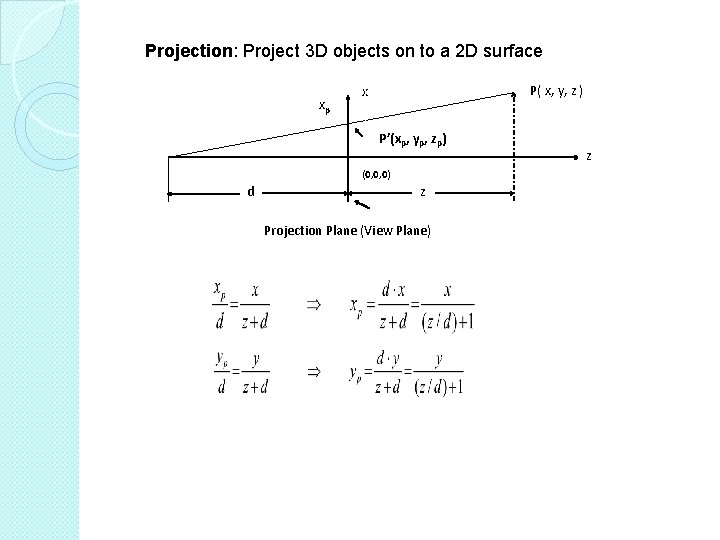Projection: Project 3 D objects on to a 2 D surface xp P( x, y, z ) x P’(xp, yp, zp) (0, 0, 0) d z Projection Plane (View Plane) z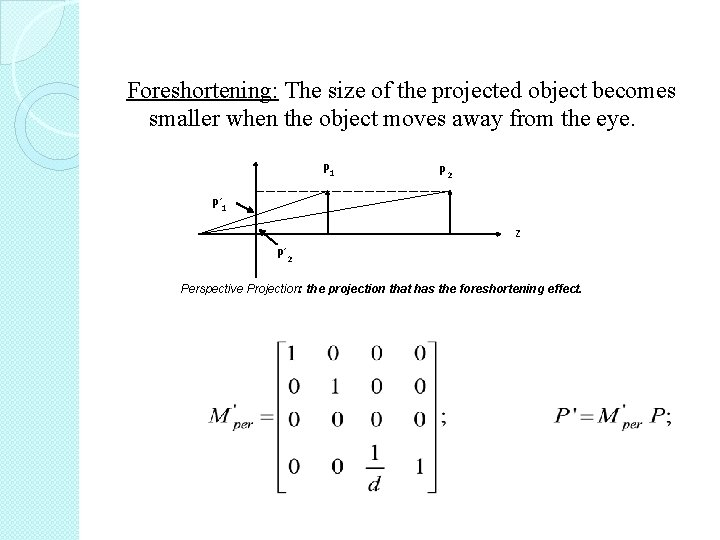Foreshortening: The size of the projected object becomes smaller when the object moves away from the eye. P 1 P 2 P’ 1 z P’ 2 Perspective Projection: the projection that has the foreshortening effect.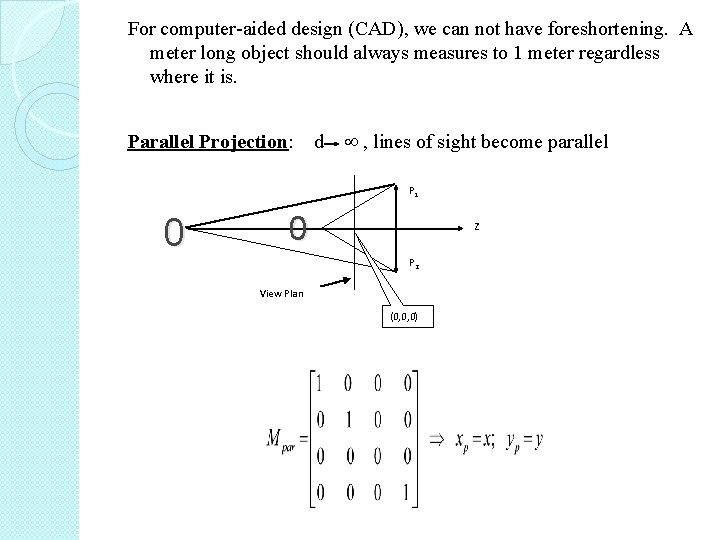For computer-aided design (CAD), we can not have foreshortening. A meter long object should always measures to 1 meter regardless where it is. Parallel Projection: d , lines of sight become parallel P 1 0 0 z P 2 View Plan (0, 0, 0)Summary � 2 D GT �Homogeneous coordinates � 3 D GT �Rigid body transformation �Affine transformation �Perspective Projection & Foreshortening �Parallel Projection